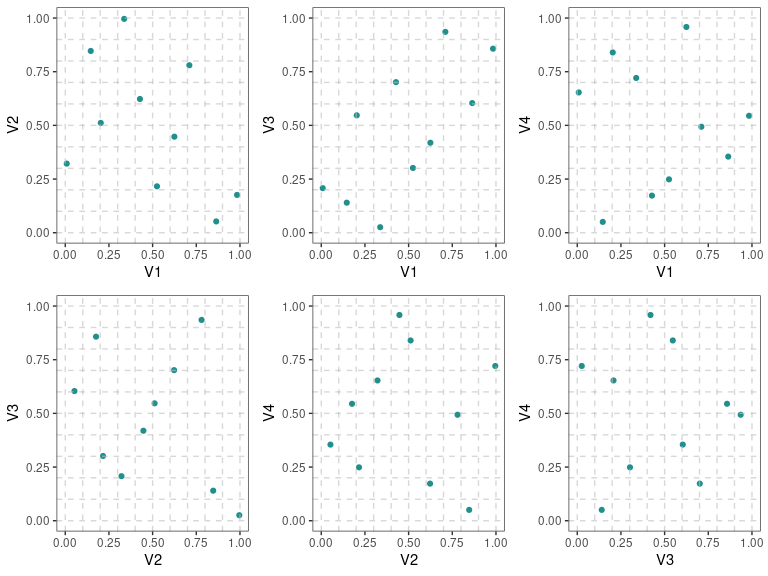## Installation

You can install:

• the latest release from CRAN with
install.packages('ggDoE')
• the development version from GitHub with
if (!require("remotes")) install.packages("remotes")
remotes::install_github("toledo60/ggDoE")

## Overview

With ggDoE you’ll be able to generate common plots used in Design of Experiments with ggplot2.

library(ggDoE)

The following plots are currently available:

The following datasets/designs are included in ggDoE as tibbles:

“Experiments: Planning, Analysis, and Optimization, 2nd Edition”

• original_epitaxial: Original epitaxial layer experiment obtain from the book
“Experiments: Planning, Analysis, and Optimization, 2nd Edition”

• aliased_design: D-efficient minimal aliasing design obtained from the article
“Efficient Designs With Minimal Aliasing by Bradley Jones and Christopher J. Nachtsheim”

## Citation

If you want to cite this package in a scientific journal or in any other context, run the following code in your R console

citation('ggDoE')
Warning in citation("ggDoE"): no date field in DESCRIPTION file of package
'ggDoE'

Warning in citation("ggDoE"): could not determine year for 'ggDoE' from package
DESCRIPTION file

To cite package 'ggDoE' in publications use:

Toledo Luna J (????). _ggDoE: Modern Graphs for Design of Experiments
with 'ggplot2'_. R package version 0.7.9,
<https://ggdoe.netlify.app>.

A BibTeX entry for LaTeX users is

@Manual{,
title = {ggDoE: Modern Graphs for Design of Experiments with 'ggplot2'},
author = {Jose {Toledo Luna}},
note = {R package version 0.7.9},
url = {https://ggdoe.netlify.app},
}

## Contributing to the package

I welcome feedback, suggestions, issues, and contributions! Check out the CONTRIBUTING file for more details.

## Examples of Plots

### Alias Matrix

Correlation matrix plot to visualize the Alias matrix

alias_matrix(design=aliased_design)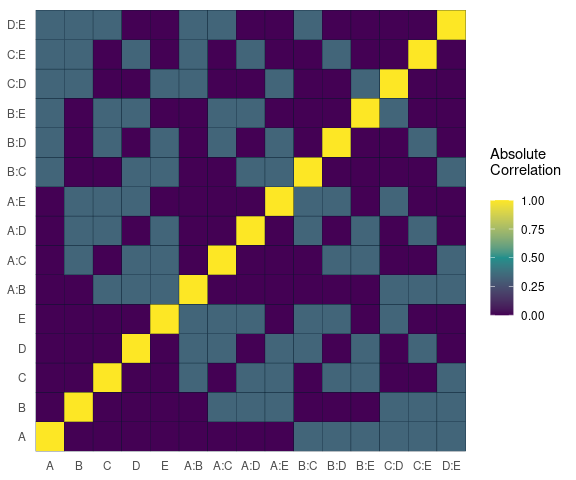### Box-Cox Transformation

model <- lm(s2 ~ (A+B+C+D),data = adapted_epitaxial)
boxcox_transform(model,lambda = seq(-5,5,0.2))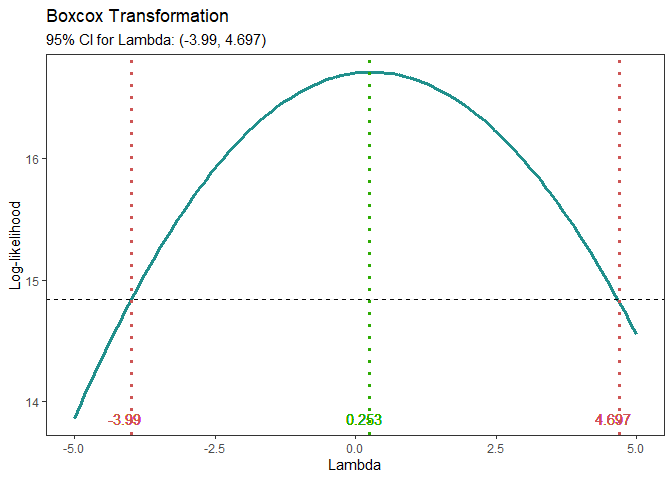### Lambda Plot

Obtain the trace plot of the t-statistics after applying Boxcox transformation across a specified sequence of lambda values

model <-  lm(s2 ~ (A+B+C)^2,data=original_epitaxial)
lambda_plot(model)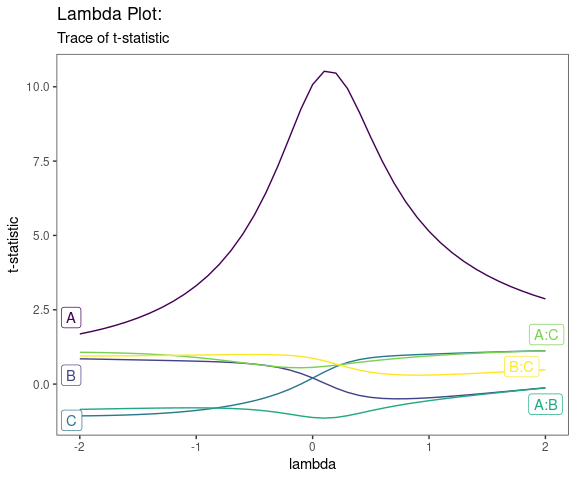lambda_plot(model, lambda = seq(0,2,by=0.1))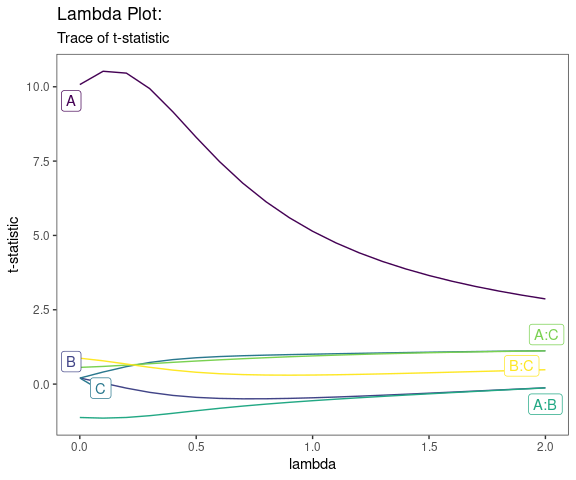### Boxplots

data <- ToothGrowth
data$dose <- factor(data$dose,levels = c(0.5, 1, 2),
labels = c("D0.5", "D1", "D2"))

gg_boxplots(data,y = 'len',x = 'dose')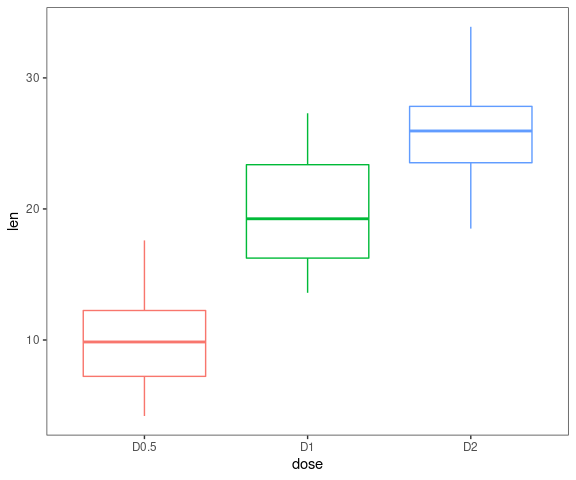gg_boxplots(data,y = 'len',x = 'dose',
group_var = 'supp',
color_palette = 'viridis',
jitter_points = TRUE)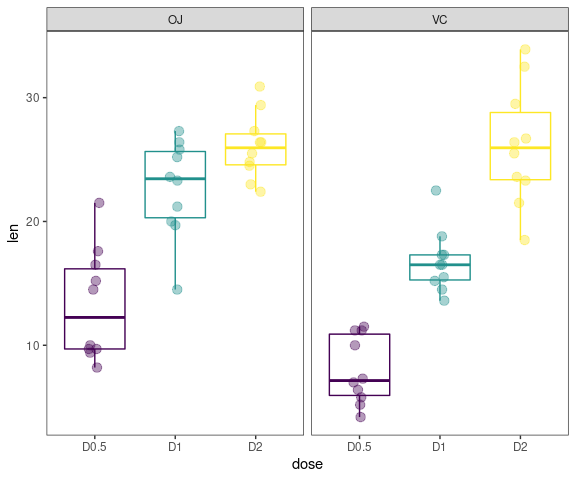### Regression Diagnostic Plots

1. Residual vs. Fitted Values
2. Normal-QQ plot
3. Scale-Location plot
4. Residual vs. Leverage
5. Cook’s Distance
6. Collinearity

The default plots are 1-4

model <- lm(mpg ~ wt + am + gear + vs * cyl, data = mtcars)
gg_lm(model,which_plots=1:6)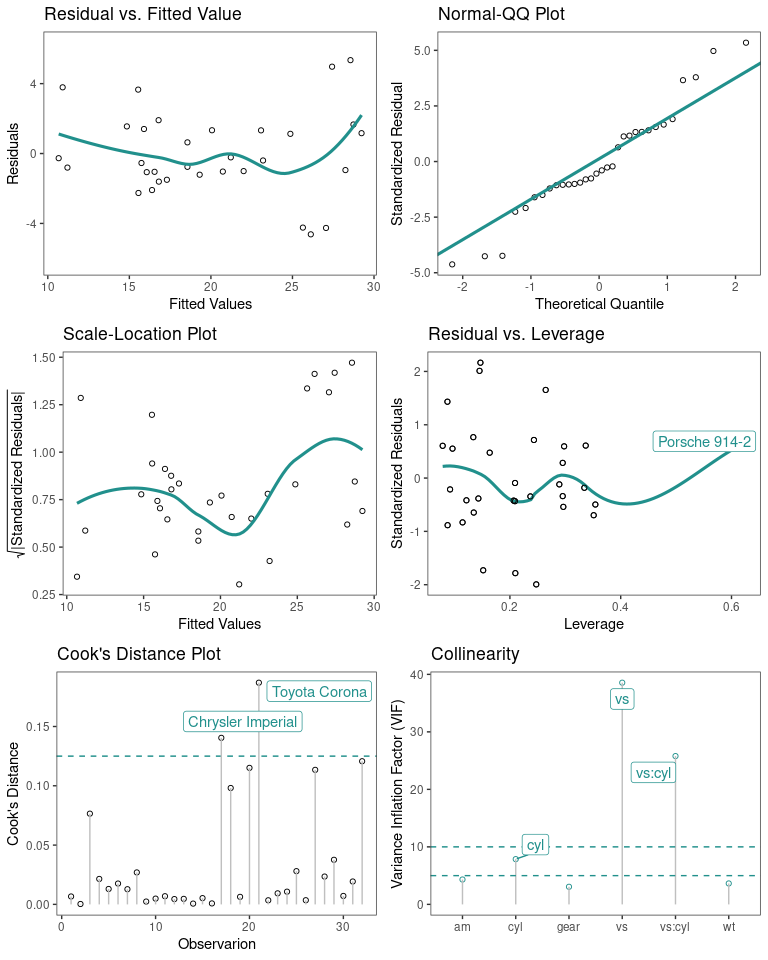### Half-Normal Plot

model <- lm(ybar ~ (A+B+C+D)^4,data=adapted_epitaxial)
half_normal(model)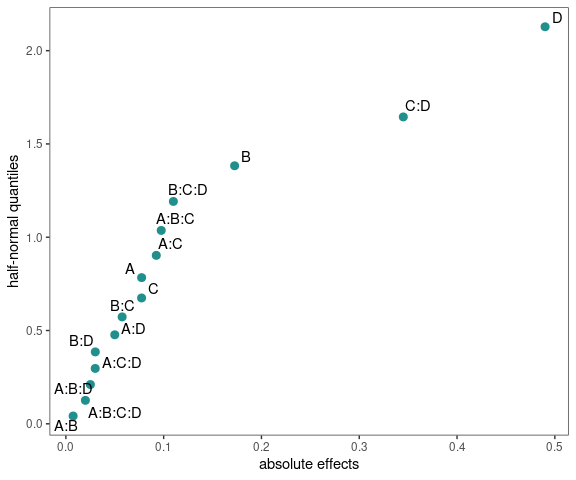half_normal(model,method='Zahn',alpha=0.1,
ref_line=TRUE,label_active=TRUE,
margin_errors=TRUE)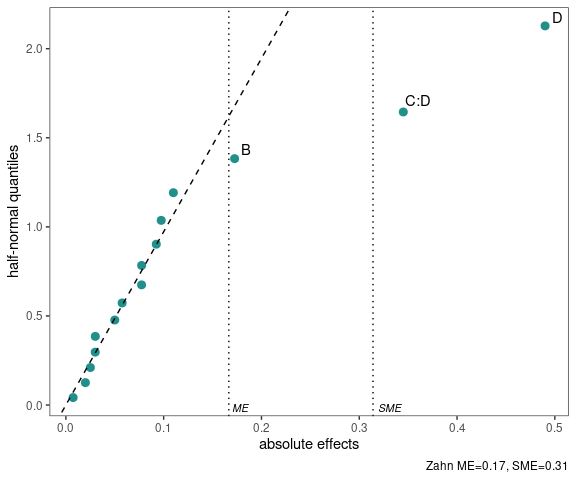### Interaction Effects Plot

Interaction effects plot between two factors in a factorial design

interaction_effects(adapted_epitaxial,response = 'ybar',
exclude_vars = c('s2','lns2'))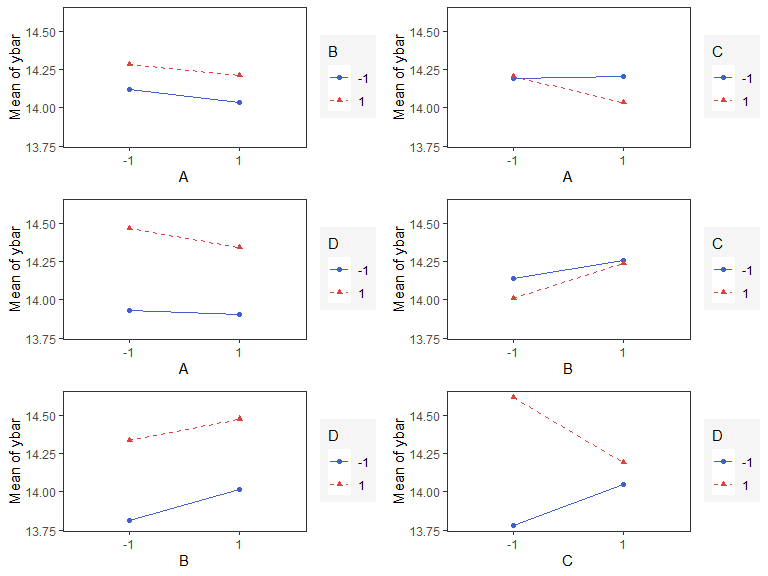interaction_effects(adapted_epitaxial,response = 'ybar',
exclude_vars = c('A','s2','lns2'),
n_columns=3)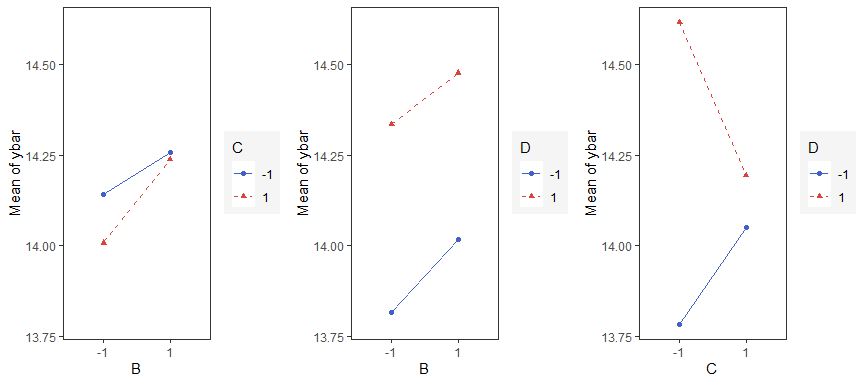### Main Effects Plots

Main effect plots for each factor in a factorial design

main_effects(original_epitaxial,
response='s2',
exclude_vars = c('ybar','lns2'))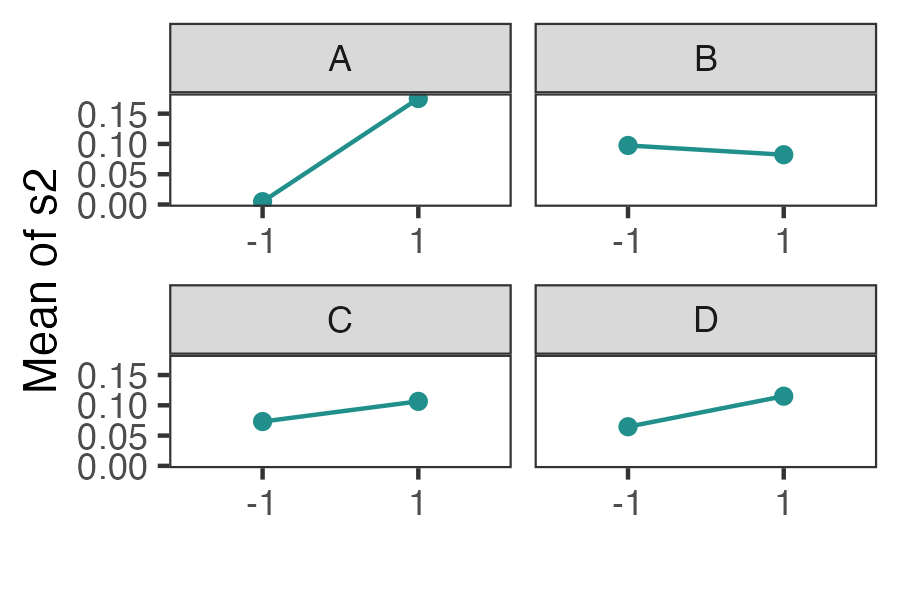main_effects(original_epitaxial,
response='s2',
exclude_vars = c('A','ybar','lns2'),
color_palette = 'viridis',
n_columns=3)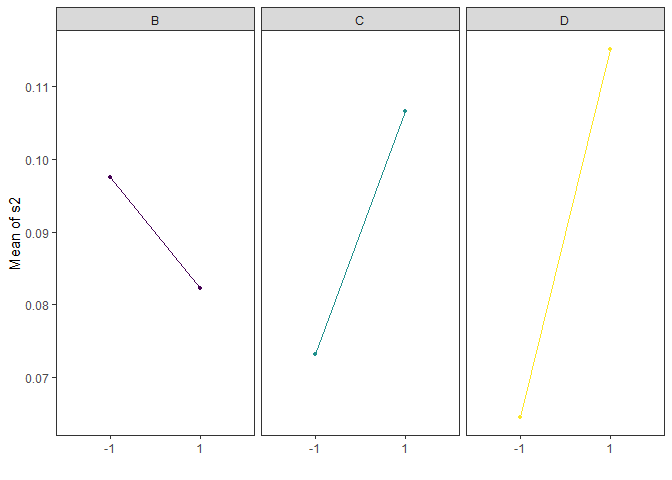### Contour Plots

contour plot(s) that display the fitted surface for an rsm object involving two or more numerical predictors

heli.rsm <- rsm::rsm(ave ~ SO(x1, x2, x3, x4),
data = rsm::heli)
gg_rsm(heli.rsm,form = ~x1+x2+x3+x4,
at = rsm::xs(heli.rsm))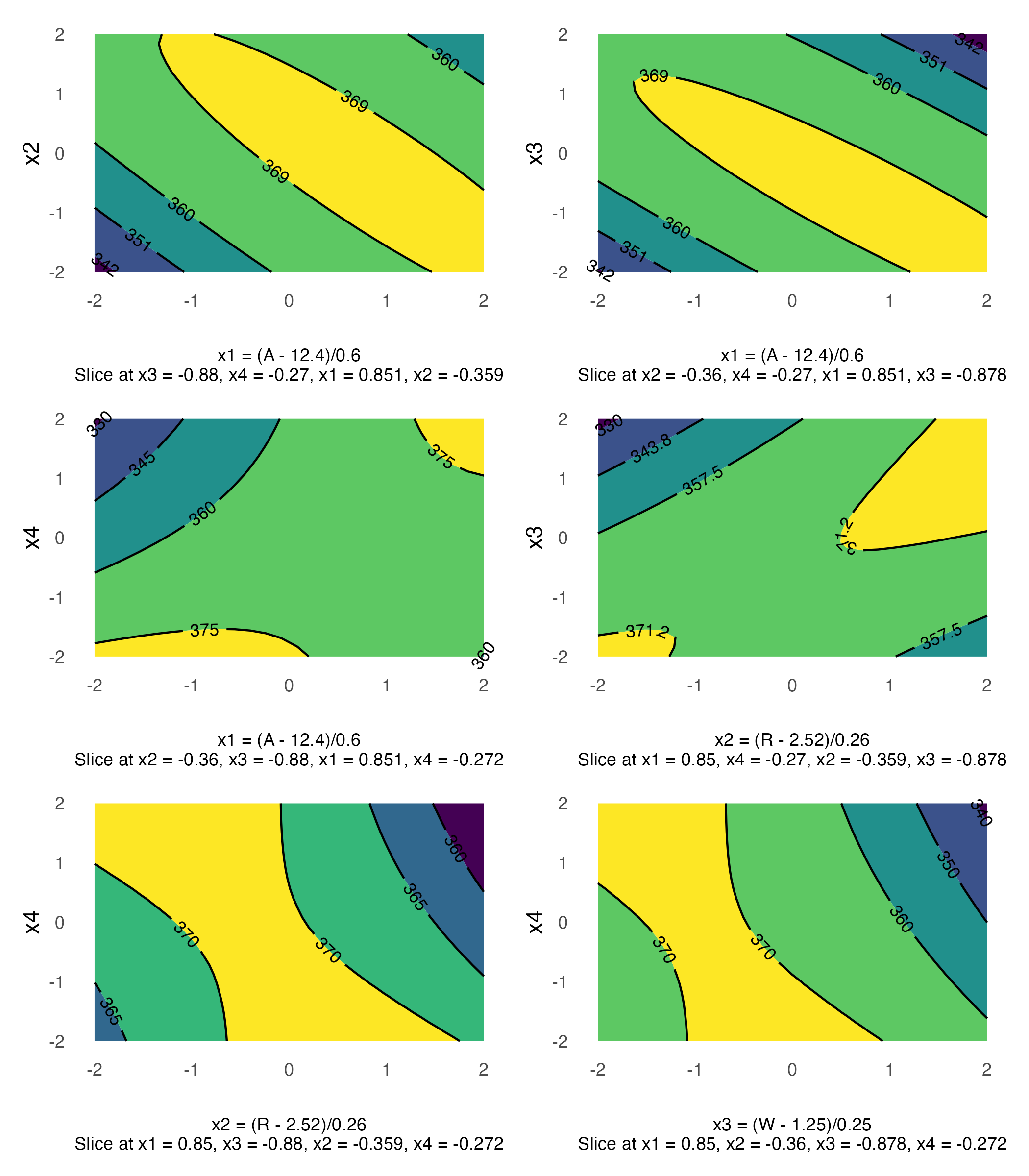gg_rsm(heli.rsm,form = ~x1+x2+x3+x4,
at = rsm::xs(heli.rsm),
filled = TRUE)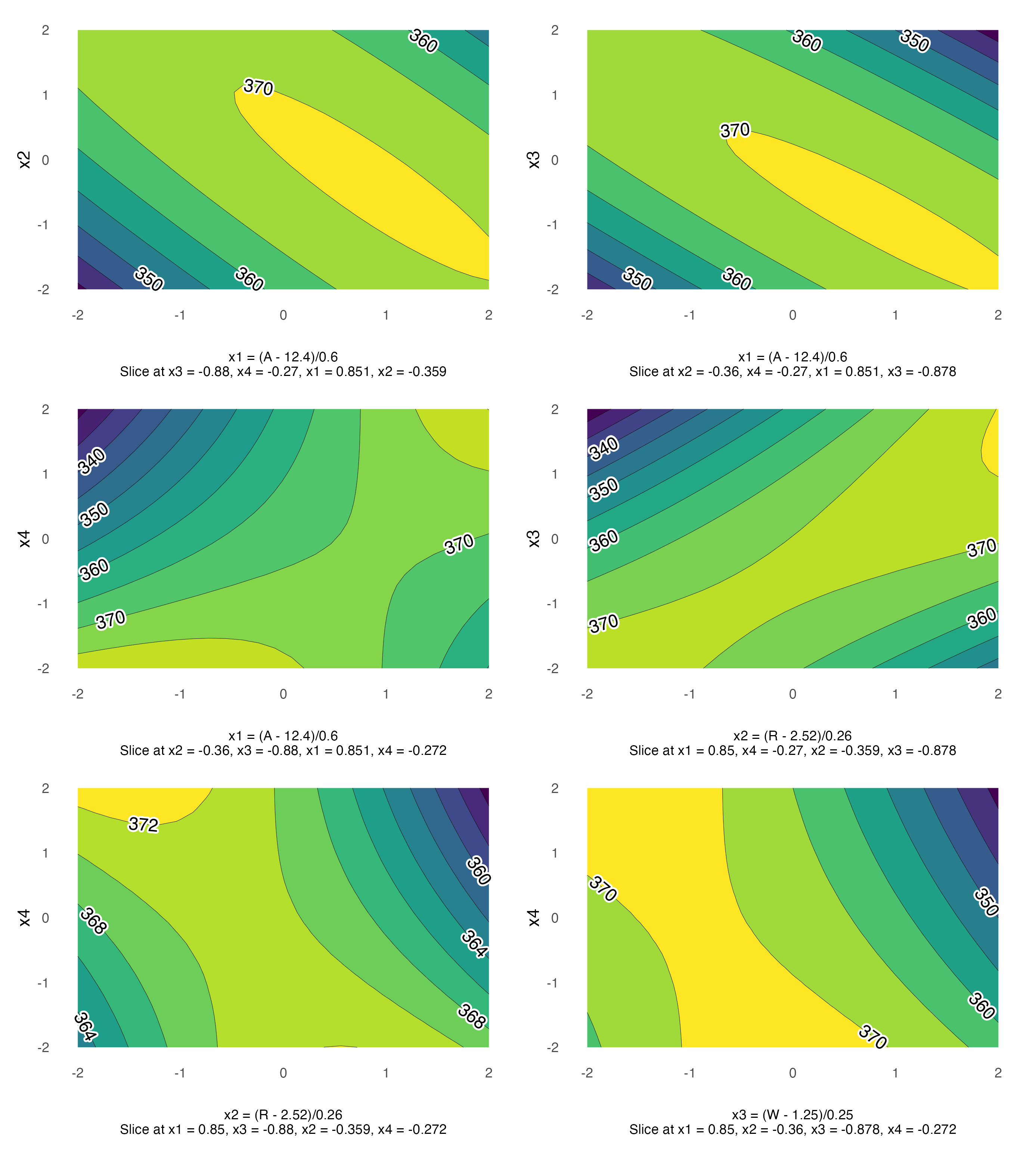### Pareto Plot

Pareto plot of effects with cutoff values for the margin of error (ME) and simultaneous margin of error (SME)

model <- lm(lns2 ~ (A+B+C+D)^4,data=original_epitaxial)
pareto_plot(model)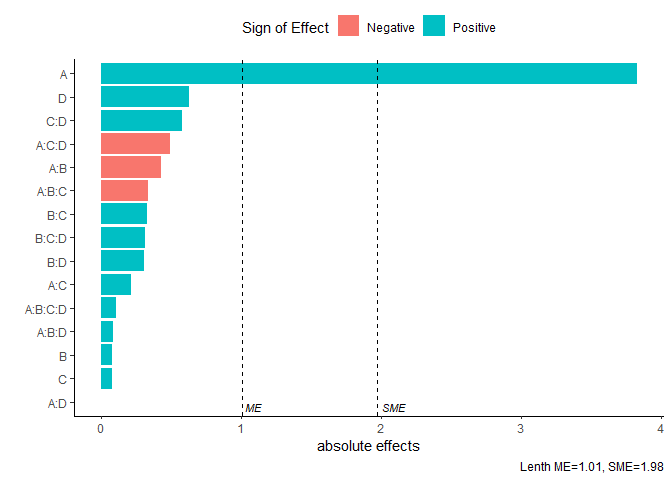pareto_plot(model,method='Zahn',alpha=0.1)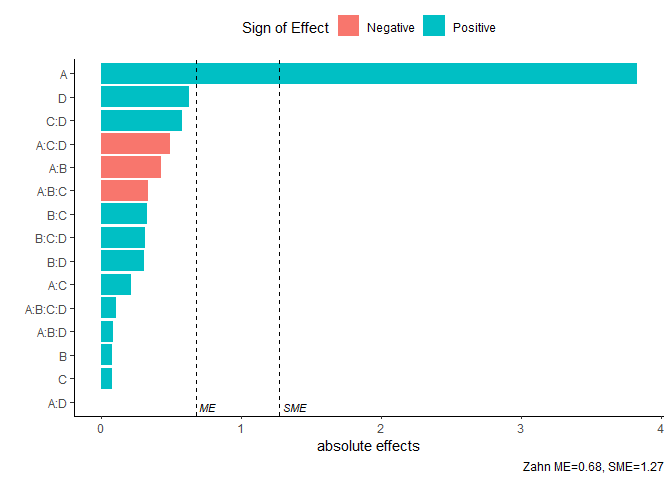### Two Dimensional Projections

This function will output all two dimensional projections from a Latin hypercube design

set.seed(10)
X <- lhs::randomLHS(n=10, k=4)
pair_plots(X,n_columns=3,grid = TRUE)